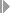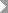Show TOC

###(09) Completed Contract Method#### Use

You can use the Completed Contract Method for:

• Sales orders

• Projects

The Completed Contract Method enables the use of conservative accounting practices because revenues and profits are only realized when the order is completed.

#### Prerequisites

Choose a results analysis method in simplified Customizing for Product Cost by Sales Order underPeriod-End ClosingResults AnalysisValuation Method.

#### Features

Until this sales order item has the status that causes the reserves and inventories to be canceled, the system updates all revenues as revenue surplus and all costs as capitalized costs.

Situation when the order is released:

• R(PA) = 0

• C(PA) = 0

• C(z) = C(a)

• R(r) = R(a)

The revenue affecting net income and the costs affecting net income are zero.

The capitalized costs are equal to the actual costs.

The revenue surplus equals the actual revenue. The revenue surplus is basically a reserve.

The capitalized costs and the revenue surplus can be transferred to FI and EC-PCA when you settle.

Situation when the order is closed:

• R(PA) = R(a)

• C(PA) = C(a)

• C(z) = 0

• R(r) = 0

#### Example

You have planned revenues of USD 200,000 and costs of USD 120,000 for your sales order.

Period 01

In period 01 you have actual costs of USD 20,000 but no revenues. In results analysis, the system calculates the following data:

• Cost of sales affecting net income of USD 0

• Revenue affecting net income of USD 0

• Capitalized costs in the amount of the actual costs (USD 20,000)

You then settle the following:

• Capitalized costs to FI and EC-PCA

• No cost of sales and no revenues to CO-PA

The following values are reported in CO-PA:

Profitability Analysis

 Revenues affecting net income 0 Cost of sales affecting net income 0 Profit 0

The income statement shows the following values:

Income Statement

 Expense Revenue Actual costs 20,000 Inventory increase Capitalized costs 20,000 20,000 20,000

Period 02

In period 02 actual costs increase to USD 80,000. You deliver to your customer and send a milestone invoice for USD 100,000. The order is partially delivered and partially billed. In results analysis, the system calculates the following data:

• Cost of sales affecting net income of zero

• Revenue affecting net income of zero

• Capitalized costs of USD 80,000

• Revenue surplus of USD 100,000

You then settle the following:

• Capitalized costs to FI and EC-PCA

• Revenue surplus to FI and EC-PCA

The following values are reported in CO-PA:

Profitability Analysis

 Revenues affecting net income 0 Cost of sales affecting net income 0 Profit 0

The income statement shows the following values:

Income Statement

 Expense Revenue Actual costs 80,000 Actual revenue 100,000 Revenue surplus 100,000 Inventory increase capitalized costs 80,000 180,000 180,000

Period 03

In period 03 actual costs increase to USD 90,000. You deliver a second amount to your customer and send a second milestone billing for USD 90,000. Total revenue is USD 190,000. The order is partially delivered and partially billed. In results analysis, the system calculates the following data:

• Cost of sales affecting net income of zero

• Revenue affecting net income of zero

• Capitalized costs of USD 90,000

• Revenue surplus of USD 190,000

You then settle the following:

• Capitalized costs to FI and EC-PCA

• Revenue surplus to FI and EC-PCA

The following values are reported in CO-PA:

Profitability Analysis

 Actual Revenues 0 Calculated cost of sales 0 Profit 0

The income statement shows the following values:

Income Statement

 Expense Revenue Actual costs 90,000 Actual revenue 190,000 Revenue surplus 190,000 Capitalized costs 90,000 280,000 280,000

Period 04

In period 04 actual costs increase to USD 130,000. You deliver the remaining goods and send the customer the final invoice for USD 10,000. Total revenue is USD 200,000. The order is now fully delivered and fully invoiced.

In results analysis, the system calculates the following:

• Cost of sales of USD 130,000

• Revenue affecting net income of USD 200,000

• Capitalized costs of zero

• Revenue surplus of zero

You then settle the following:

• Cost of sales to CO-PA

• Revenues to CO-PA

• The cancellation of the capitalized costs and the revenue surplus to FI and EC-PCA

The following values are reported in CO-PA:

Profitability Analysis

 Actual revenues 200,000 Calculated cost of sales 130,000 Profit 70,000

The income statement shows the following values:

Income Statement

 Expense Revenue Actual costs 130,000 Actual revenue 200,000 Profit 70,000 200,000 200,000

The order has a total profit of USD 70,000.